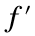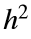# Problem 46053. Construct finite difference approximations of derivatives

In solving a differential equation with a finite-difference method, one computes derivatives with various combinations of the function's values at chosen grid points. For example, the forward difference formula for the first derivative iswhere j is the grid index and h is the spacing between points. The systematic approach for deriving such formulas is to use Taylor series. In the example above, one can writeThen solving forand neglecting terms of orderand higher givesBecause the exponent on h in the last term is 1, the method is called a first order method.
Write a function that takes the order n of the derivative and a vector terms indicating the terms to use (based on the number of grid cells away from the point in question) and produces a vector of coefficients, the order of the error term, and the numerical coefficient of the error term. In the above example, n = 1 and terms = [1 0], and
coeffs = [1 -1]
errOrder = 1
errCoeff = -0.5;

### Solution Stats

100.0% Correct | 0.0% Incorrect
Last Solution submitted on Jan 16, 2021

### Community Treasure Hunt

Find the treasures in MATLAB Central and discover how the community can help you!

Start Hunting!